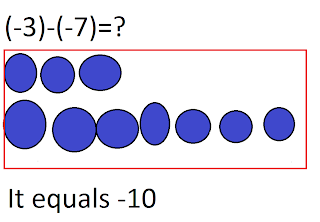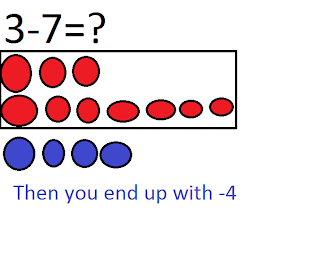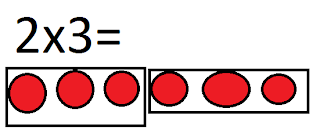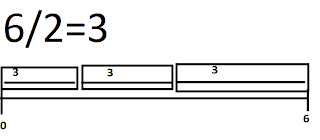## Wednesday, March 23, 2011

### Kevin's Big Book Of Intergers

Chapter 1:

Integers are basically positives (+), negatives (-), and zeropairs.What I remembered:
-Integers can be shown on a number line.
-The rhyme "When subtracting something that isn't, there use a zero pair"

The * question we have to do:
(-3)-(-7)=-3-7=\
3-7=3+7=Chapter 2 Multiplying Integers:
Examples:
(+2)x(+3)=6It basically means you have 2 groups of 3.

2x(-3)=(-6)When you have a negative on the right, it is just the same thing except the number is negative
(remember + and a - make a -, a - and a - make a +)

(-2)x(+3)=-6-2x(-3)=6Chapter 3:
Dividing Integers

Partitive Division:
Partitive division is how many groups of something are in something. We use a number line to show this.Quotative Division:
This really just means "How do you share 6 with 2?"The multiplicative inverse can helps us solve division questions because if we divide 6 by 2 we have 3. Now 3, we know that 3x2 equals 6 or 2x3 equals 6.

Chapter 4 :Order of Operations with Integers

(+5) x (-3) + (-6) ÷ (+3)=
[(+5) x (-3)] + [(-6) ÷ (+3)]=
(-15) + (-2) = -17

Use BEDMAS :)!